Next: Results on the Structure Up: Applications/New Directions Previous: Li and Milner's Structure

## The Cancellation Problem

The cancellation problem is a long standing open problem by G. Birkhoff. If we denote the set of all order-preserving maps from the ordered set Q to the ordered set P by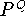(which naturally is an ordered set under the pointwise order), the problem is the following:   Let P,Q,R be finite ordered sets. Does the fact thatis isomorphic to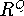imply that P is isomorphic to R? The finiteness assumption is needed, as the examples 4 and 5 in  on page 21 show. For an overview on such arithmetic topics in ordered sets and the cancellation problem in particular, cf. Jónsson's survey .
Uniqueness of cores, which was discussed in ,  and  yields a new insight, though it is not clear how far Proposition 5.5 can be pushed (cf. open question 8).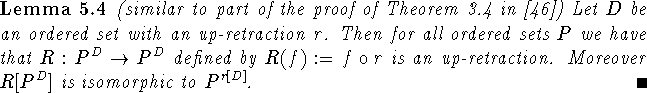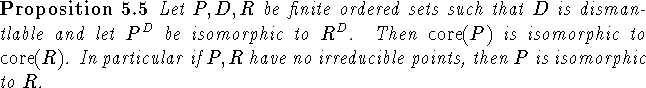Proof: By Lemma 5.4 we have that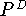can be dismantled via comparative retractions to a subset that is isomorphic to P. (This also answers question (2) on p.54 in .) Thuscan be dismantled via comparative retractions to a subset that is isomorphic to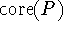. By the uniqueness of the core, we infer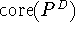is isomorphic to. Similarly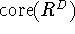is isomorphic to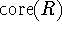, and the isomorphic setsand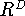naturally must have isomorphic cores. \

Bernd.S.W.Schroder